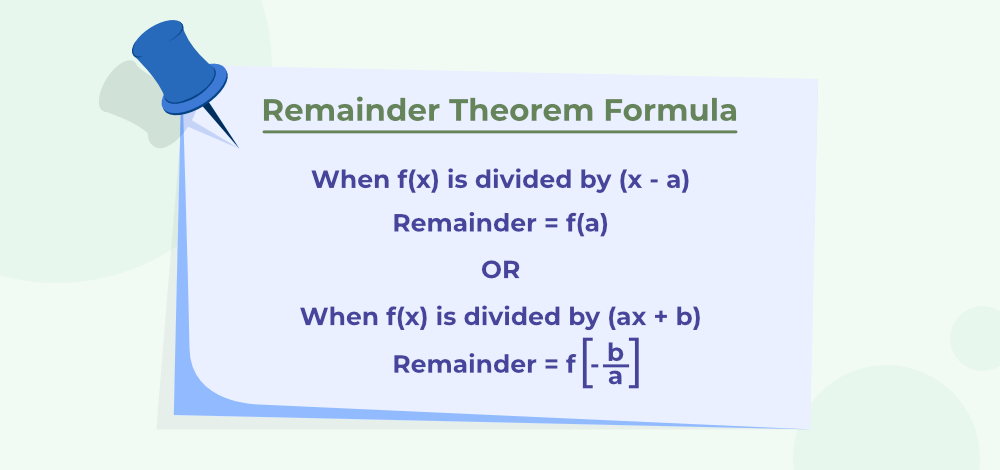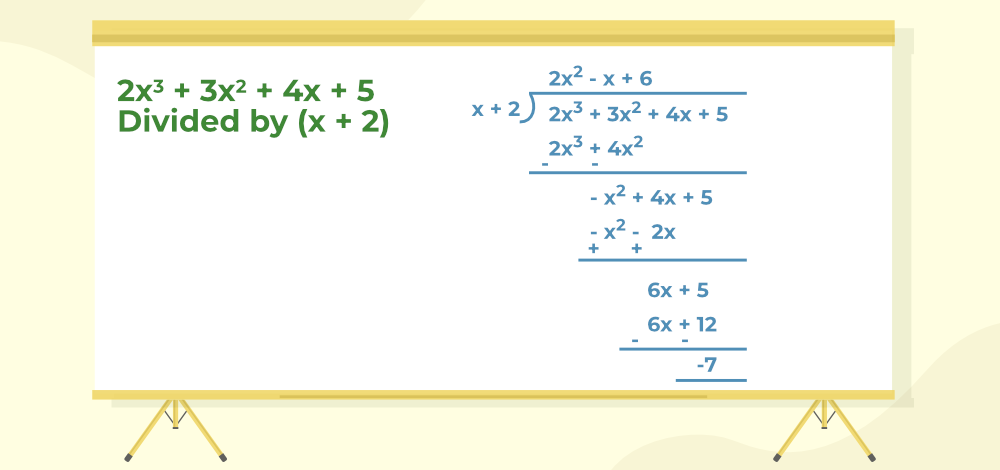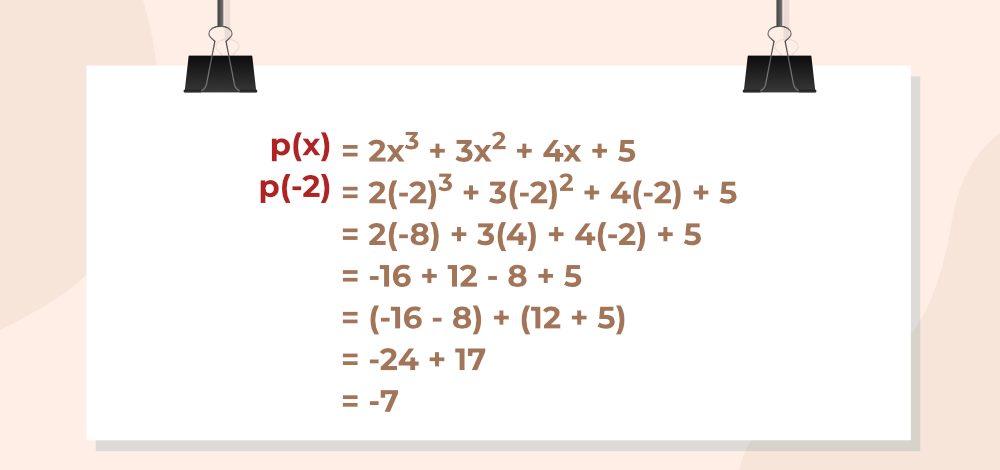GFG App
Open AppBrowser
Continue

Remainder theorem is the basic theorem used in mathematics which is used to find the remainder of any polynomial when it is divided by a linear polynomial. Suppose for any given polynomial f(x) if it is divided by x-a then its reminder is always f(a). Remainder theorem works on the principle of Euclidean division theorem. Linear theorem works only when the division is linear and it fails for other types of divisors. Now let’s learn about the Reminder theorem, its proof, and others in detail in this article.

## Remainder Theorem Definition

Remainder theorem states that for any polynomial whose degree is greater than or equal to 1 if it is divided by a linear polynomial q(x) such that q(x) = x-a, the remainder of this division is always equal to q(a). This theorem is very helpful in finding the remainder of the polynomial without actually performing the division. The remainder theorem can be easily expressed as, for any polynomial p(x) { degree greater than or equal to 1} if divided by any linear polynomial s(x) = x-a the reminder is always p(a) such that,

p(x)/s(x) = q(x) + r(x)

where,
q(x) is the quotient,
r(x) is the remainder which is equal to r(a)

## Remainder Theorem Formula

For any polynomial function f (x) when divided by the linear polynomial (x-a) then the remainder is always equal to f (a).

f (x) = (x-a) × q (x) + r (x)

where,
r(x) is the remainder of the polynomial

The image given below express the remainder theorem.## Factor Theorem

Factor Theorem is also the basic theorem of mathematics which is considered the reverse of the remainder theorem. This is generally used the find roots of polynomial equations.

This theorem states that for any polynomial p(x) if p(a) = 0 then x-a is the factor of the polynomial p(x).

## Remainder Theorem Proof

For any polynomial g(x) the remainder theorem can easily be proved as,

Let g(x) be a polynomial with a degree of 1 or greater than 1. Suppose that when g(x) is divided by (x – b), the quotient is q(x) and the remainder is r(x), i.e.

g(x) = (x – b) q(x) + r(x)

Since the degree of x – b is 1 and that of r(x) is less than that of x – b, the degree of r(x) = 0. This means that r(x) is constant. Thus, we can write the above equation as

g(x) = (x – b) q(x) + r

In particular, if x = b, then the equation becomes,

p(b) = (b – b) q(b) + r

p(b) = (0) q(b) + r

p(b) = r

Hence, Proved.

## Dividing a Polynomial by a Non-Zero Polynomial

For dividing a polynomial with a non-zero polynomial use the steps given below,

Step 1: Arrange both the polynomial dividend and divisor in the descending order of their degree.

Step 2: The first term of the dividend is divided by the first term of the divisor to produce the quotient,

Step 3: After subtracting the first term arrange the remainder and copy the second term of the dividend and start dividing the remaining term as discussed above.

Step 4: Repeat these steps until the degree of the dividend polynomial is less than the divisor. In the end, only the remainder is left.

## Remainder Theorem of Polynomial

How to use the Remainder theorem? can easily be explained with the help of the example given below.

Example: Divide 2x3 + 3x2 + 4x + 5 by x + 2

Solution:

Given,

Dividend = p(x) = 2x3 + 3x2 + 4x + 5

Divisor = s(x) = (x + 2)Here,

Quotient = q(x) =2x2 – x + 6

Remainder = r(x) = -7

Verification:

Given,

Divisor = (x + 2)

Let x + 2 = 0

x  = -2

According to Remainder Theorem, substituting x = -1 in p(x),Now, Remainder  = p(-2)

Thus, Remainder Theorem is verified.

### Alternate Method

We know that any polynomial p(x) can easily be written as,

p(x) = (x – a)·q(x) + r

Taking x = a in the above equation we get only the remainder,

similarly, for any p(x)

p(x) = (x+2).q(x) + r

if we put x = -2 we get the remainder of the above equation.

Thus, p(-2) is the remainder.

Hence proved.

## Euler Remainder Theorem

For any two co-prime positive integers n and X, Euler’s theorem  states that,

Xφ(n) = 1 (mod n)

where,
φ(n) is called Euler’s function and its value is given as,

φ(n) = n (1-1/a)×(1-1/b)(1-1/c)

where,
n is a natural number, and n = ap × bq × cr

where,
a, b, c are prime factors of n and p, q, r are positive integers.

Example: Find the Euler function of 21

Solution:

The factors of 21 are,

21 = 7×3

φ(21) = 21 (1 – 1/7)(1 – 1/3)

= 21 × 6/7 × 2/3

= 12

Thus, the Euler function of 21 is 12

## Differences between the Remainder Theorem and Factor Theorem

Remainder Theorem and Factor Theorem are the basic theorems in mathematics and the basic difference between them is stated in the table given below,

## Solved Examples on Remainder Theorem

Example 1: Find the remainder when p(x) = x4 – x3 + x2 – 2x + 1 is divided by g(x) = x – 2.

Solution:

Given,

p(x) = x4 – x3 + x2 – 2x + 1

g(x) = x-2

Using Remainder theorem,

p(2) = (2)4 – (2)3 + (2)2 + 2(2) + 1

= 17

Thus, the remainder when p(x) is divided by g(x) then we get remainder as, 17

Example 2: Find the root of the polynomial x2 – 5x + 4

Solution:

We know that if for any p(x) we get p(a) = 0, then x-a is the factor of p(x) or a is the root of equation.

Given,

f(x) = x2 – 5x + 4

By hit and try method.

f(4) = 42 – 5(4) + 4

f(4) = 20 – 20

= 0

So, (x – 4) must be a factor of x2 – 5x + 4

Example 3: Find the remainder when t3 – 2t2 + 4t + 5 is divided by t – 1.

Solution:

Given,

p(t) = t3 – 2t2 + 4t + 5

g(t) = t – 1

Using Remainder theorem,

g(1) = (1)3 – 2(1)2 + 4 + 5

= 8

By the Remainder Theorem, 8 is the remainder when t3 – 2t2 + 4t + 5 is divided by t – 1

Example 4: Find the remainder when x3 x2 + 2 is divided by x – 2.

Solution:

Given,

p(x) = x3 x2 + 2

g(x) = x-2 = 0

x = 2

Using Remainder theorem,

g(2) = (2)3 – (2)2 + 2

= 6

By the Remainder Theorem, 6 is the remainder when x3 – x2 + 2 is divided by x – 2

Example 5: Find the root of the polynomial 3x2 – 7x + 2

Solution:

We know that if for any p(x) we get p(a) = 0, then x-a is the factor of p(x) or a is the root of equation.

Given,

f(x) = 3x2 – 7x + 2

By hit and try method.

f(2) = 3(2)2 – 7(2) + 2

f(2) = 12 -14 + 2

= 0

So, (x – 2) must be a factor of 3x2 – 7x + 2 and 2 is the root of 3x2 – 7x + 2

## FAQs on Remainder Theorem

### Question 1: What is the Remainder Theorem?

According to remainder theorem for any polynomial p(x) (whose degree is greater than equal to 1) when divided by the linear polynomial (x – a), the remainder is always p(a).

### Question 2: Who Invented the Remainder Theorem?

The credit for inventing remainder theorem goes to Chinese mathematician Sun Zi and the it was completed by Qin Jiushao in 1247.

### Question 3: What is the use of the Factor Theorem?

Factor theorem is used to find the factors of the given polynomial. For any polynomial f(x), x-a is the factor of the polynomial f(x) only when, f(a) is zero.

### Question 4: What are the Applications of the Remainder Theorem Formula?

Remainder theorem is widely used to find the remainder of the polynomial without actually performing the long division and remainder theorem along with factor theorem is widely used to solve the polynomial equation.